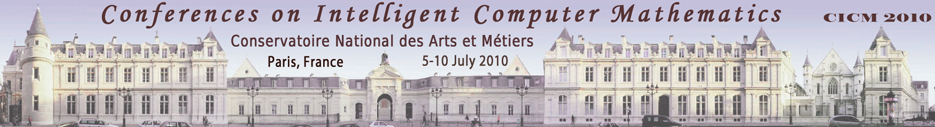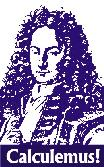### 17th Symposium on the Integration of Symbolic Computation and Mechanised Reasoning Calculemus 2010 Paris, France    6th-7th July 2010Accepted Papers

The list of accepted papers below is ordered alphabetically by first author surname.

Links to preprints (on http://ArXiv.org) and to the final published articles (on http://www.springerlink.com) will be added as they become available.

The full proceedings of the conference are available from Springer (LNAI 6167). You can find information about it at http://www.springeronline.com/978-3-642-14127-0 or access the online version at http://www.springerlink.com/content/978-3-642-14127-0/

• Formal Proof of SCHUR Conjugate Function
Franck Butelle, Florent Hivert, Micaela Mayero and Frédéric Toumazet
Abstract: The main goal of our work is to formally prove the correctness of the key commands of the SCHUR software, an interactive program for calculating with characters of Lie groups and symmetric functions. The core of the computations relies on enumeration and manipulation of combinatorial structures. As a first "proof of concept", we present a formal proof of the conjugate function, written in C. This function computes the conjugate of an integer partition. To formally prove this program, we use the Frama-C software. It allows us to annotate C functions and to generate proof obligations, which are proved using several automated theorem provers. In this paper, we also draw on methodology, discussing on how to formally prove this kind of program.
• Symbolic Domain Decomposition
Jacques Carette, Alan Sexton, Volker Sorge and Stephen Watt
Abstract: Decomposing the domain of a function into parts has many uses in mathematics. A domain may naturally be a union of pieces, a function may be defined by cases, or different boundary conditions may hold on different regions. For any particular problem the domain can be given explicitly, but when dealing with a family of problems given in terms of symbolic parameters, matters become more difficult. This article shows how hybrid sets, that is multisets allowing negative multiplicity, may be used to express symbolic domain decompositions in an efficient, elegant and uniform way, simplifying both computation and reasoning. We apply this theory to the arithmetic of piecewise functions and symbolic matrices and show how certain operations may be reduced from exponential to linear complexity.
• A Formal Quantifier Elimination for Algebraically Closed Fields
Cyril Cohen and Assia Mahboubi
Abstract: We prove formally that the first order theory of algebraically closed fields enjoys quantifier elimination, and hence is decidable. This proof is organized in two modular parts. We first reify the first order theory of rings and prove that quantifier elimination leads to decidability. Then we implement an algorithm which constructs a quantifier free formula from any first order formula in the theory of ring. If the underlying ring is in fact an algebraically closed field, we prove that the two formulas have the same semantic. The algorithm producing the quantifier free formula is programmed in continuation passing style, which leads to both a concise program and an elegant proof of semantic correctness.
• Computing in Coq with Infinite Algebraic Data Structures
Cesar Dominguez and Julio Rubio
Abstract: Computational content encoded into constructive type theory proofs can be used to make computing experiments over concrete data structures. In this paper, we explore this possibility when working in Coq with chain complexes of infinite type (that is to say, generated by infinite sets) as a part of the formalization of a hierarchy of homological algebra structures.
• Formally Verified Conditions for Regularity of Interval Matrices
Ioana Pasca
Abstract: We propose a formal study of interval analysis that concentrates on theoretical aspects rather than on computational ones. In particular we are interested in conditions for regularity of interval matrices. An interval matrix is called regular if all scalar matrices included in the interval matrix have non-null determinant and it is called singular otherwise. Regularity plays a central role in solving systems of linear interval equations. Several tests for regularity are available and widely used, but sometimes rely on rather involved results, hence the interest in formally verifying such conditions of regularity. In this paper we set the basis for this work: we define intervals, interval matrices and operations on them in the proof assistant Coq, and verify criteria for regularity and singularity of interval matrices.